## 1 Introduction

One of the important discoveries in substructural logic is the decomposition of the intuitionistic implication $$\phi \Rightarrow \psi$$ using the linear implication $$\multimap$$ and the exponential modality !. This discovery was studied by Girard through his linear logic, which brought many new ideas and perspectives to logic and programming language semantics.

Inside linear logic proofs, propositions with the exponential modality $$!\phi$$ can be freely copied or discarded. Later, it was realized that by adding a copy limit to the exponential modality, like $$!_r\phi$$, linear logic gains fine control of assumption usage. This idea was first implemented in bounded linear logic , and studied in connection with implicit complexity theory [4, 14]. Indexed exponential modalities $$!_r$$ were then used in wider context: resource management in programming languages [3, 7, 8, 20, 23] and control of sensitivity in the metric semantics of programs [5, 21].

The categorical structure corresponding to the exponential modality ! was studied by various researchers, and it was identified as a categorical structure called linear exponential comonad . One of the celebrated results about linear exponential comonads is that any symmetric lax monoidal adjunction:

yields a linear exponential comonad $$L\circ R$$, and every linear exponential comonad D arises in this way - for $$\mathbb {D}$$ take the category of Eilenberg-Moore coalgebras of D.

The categorical structure corresponding to the indexed exponential modality $$!_r$$ has been proposed as exponential action  and graded linear exponential comonad ; they are two different presentations of the same data. Compared to linear exponential comonads, however, categorical understanding of graded linear exponential comonads is not well-established. The aim of this paper is to contribute to this point. Concretely speaking, we show the following categorical results about graded linear exponential comonads:

• We give a new concise formulation of graded linear exponential comonads as vertical monoid homomorphisms from multiplicative monoids of semirings to the composition monoids of symmetric lax monoidal endofunctors. This formulation is given in a rather complex multi-double category of symmetric monoidal categories. The slogan is “to represent a complex structure in a simple category as a simple structure in a complex category”.

• In the multi-double category, vertical monoid homomorphisms themselves can be seen as monoids. By considering actions of such monoids, we obtain the concept of graded comonoid-coalgebras. They are an extension of Eilenberg-Moore coalgebras to graded linear exponential comonads, and the category of graded comonoid-coalgebras provides a resolution of graded linear exponential comonads by a symmetric lax monoidal adjunction plus a twist.

## 2 Related Work

Graded linear exponential comonads were first introduced as exponential actions in , and an equivalent definition was given in . This paper adopts the latter definition as the starting point of study. These papers also consider linear type systems with an indexed exponential modality $$!_r\phi$$, which is directly interpreted by a graded linear exponential comonad. This paper, however, focuses only on the categorical axiomatics of the indexed exponential modality, and omit its syntactic theory. In , Breuvart and Pagani gave a construction of graded linear exponential comonads from a set of data called stratification. They derived various graded linear exponential comonads on the category of sets and binary relations and the category of coherence spaces. Structures close to, but different from, graded linear exponential comonads were considered in the categorical semantics of the following calculi: INTML for interactive computation , coeffect calculus  and bounded affine types system .

The multicategory of symmetric lax monoidal multifunctors is related to the 2-multicategory of T-algebras for a pseudo-commutative 2-monad T . Hyland and Power studied multifunctors that are symmetric strong monoidal in each argument, while in this paper we weaken “strong” to “lax”. Yet, we think that by suitably extending their theory, the symmetric lax monoidal multifunctors can also be given in the language of 2-monad theory.

Monoids in the multicategory $$\mathbf {MSMC}_l$$ in Sect. 5 are similar to the distributivity studied in , where Laplaza considered two symmetric non-strict monoidal structures together with a colax distributivity between them. On the other hand, in this paper, we consider a strict monoidal structure on top of the underlying symmetric (non-strict) monoidal structure, and a lax distributivity between them.

Preliminaries For symmetric monoidal categories and symmetric lax monoidal functors, see . In a symmetric monoidal category $$\mathbb {C}$$, by $$\iota :\mathbf {I}\otimes \mathbf {I}\rightarrow \mathbf {I}$$ we mean the isomorphism $$\lambda _{\mathbf {I}} = \rho _\mathbf {I}$$, and by $$\tau :(A\otimes B)\otimes (C\otimes D)\rightarrow (A\otimes C)\otimes (B\otimes D)$$ we mean the symmetry swapping the second and third component of the tensor product. For functors $$F_i:\prod _{j=1}^{m_i}\mathbb {C}_{i,j}\rightarrow \mathbb {D}_i$$ where $$1\le i\le n$$, we define $$F_1\times \cdots \times F_n$$ to be the composite functor $$\textstyle \prod _{1\le i\le n,1\le j\le m_i}\mathbb {C}_{i,j}\rightarrow \prod _{i=1}^n(\prod _{j=1}^{m_i}\mathbb {C}_{i,j})\rightarrow \prod _{i=1}^n\mathbb {D}_i,$$ whose codomain is the product category without the nesting of products.

In this paper, comonads are graded by a partially ordered semiring. It is a tuple $$(R,\le ,0,+,1,*)$$ such that $$(R,0,+,1,*)$$ is a unital semiring (not necessarily commutative) and $$+,*$$ are monotone in each argument w.r.t. the partial order $$\le$$. The partially ordered monoids of additive and multiplicative parts of R are denoted by $$R^+=(R,\le ,0,+)$$ and $$R^*=(R,\le ,1,*)$$, respectively.

Let $$\mathbb {C},\mathbb {D}$$ be symmetric monoidal categories. We write $$\mathbf {SMC}_l(\mathbb {D},\mathbb {C})$$ for the category of symmetric lax monoidal functors and monoidal natural transformations between them. The following pointwise extension of the tensor unit and tensor product on $$\mathbb {C}$$ extends to a symmetric monoidal structure on $$\mathbf {SMC}_l(\mathbb {D},\mathbb {C})$$:

\begin{aligned} \dot{\mathbf {I}}(D)=\mathbf {I},\quad (F\mathbin {\dot{\otimes }}G)(D)=FD\otimes GD. \end{aligned}

(We note that the symmetry in $$\mathbb {C}$$ is used to make $$F\mathbin {\dot{\otimes }}G$$ a symmetric lax monoidal functor.) Below by $$[\mathbb {D},\mathbb {C}]_l$$ we mean the symmetric monoidal category $$(\mathbf {SMC}_l(\mathbb {D},\mathbb {C}),\dot{\mathbf {I}},\mathbin {\dot{\otimes }})$$ of symmetric lax monoidal functors and monoidal natural transformations between them.

Fix a partially ordered semiring $$(R,\le ,0,+,1,*)$$. We introduce the main subject of this study, R-graded linear exponential comonad. This concept first appeared in [3, Definition 13] under the name exponential action. We adopt the following definition [7, Sect. 5.2], which is equivalent to the exponential action:

### Definition 1

An R-graded linear exponential comonad on a symmetric monoidal category $$\mathbb {C}$$ is a tuple $$(D,w,c,\epsilon ,\delta )$$ where

• $$D:(R,\le )\rightarrow \mathbf {SMC}_l(\mathbb {C},\mathbb {C})$$ is a functor. Below we write $$m_{r}:\mathbf {I}\rightarrow D(r)(\mathbf {I})$$ and $$m_{r,A,B}: D (r) (A) \otimes D (r) (B) \rightarrow D (r) (A\otimes B)$$ for the symmetric lax monoidal structure of D(r).

• $$(D,w,c):R^+\rightarrow [\mathbb {C},\mathbb {C}]_l$$ is a symmetric colax monoidal functor.

• $$(D,\epsilon ,\delta ):R^*\rightarrow (\mathbf {SMC}_l(\mathbb {C},\mathbb {C}),\mathrm{Id},\circ )$$ is a colax monoidal functor.

They satisfy four equational axioms in Fig. 1. Moreover, we say that D is an R-twist if Dr is strong monoidal for each $$r\in R$$, and $$(D,\epsilon ,\delta )$$ is a strict monoidal functor (hence $$D1=\mathrm{Id}$$ and $$D(r*r')=Dr\circ Dr'$$).

When fully expanded, a graded linear exponential comonad specifies one functor $$D:(R,\le )\rightarrow [\mathbb {C},\mathbb {C}]$$ and 6 natural transformations: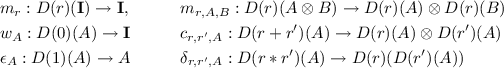satisfying more than 20 equational axioms.

### Example 1

Let $$\mathbb {C}$$ be a cartesian closed category. We take a partially ordered monoid $$R^\times =(R,\le ,1,\times )$$ such that $$(R,\le )$$ is a join semilattice and $$\times$$ preserves joins in both arguments. This condition makes the tuple $$R=(R,\le ,\bot ,\vee ,1,\times )$$ a partially ordered semiring. We also take a lax monoidal functor $$G:R^\times \rightarrow \mathbb {C}$$. Then the functor $$D:(R,\le )^{op}\rightarrow [\mathbb {C},\mathbb {C}]$$ defined by $$DrA=Gr\Rightarrow A$$ extends to an $$R^{op}$$-graded linear exponential comonad on $$\mathbb {C}$$ (here $$R^{op}$$ is the order-opposite of R).

### Example 2

Continuing the previous example, let $$R=(D,\le ,\bot ,\vee ,\top ,\wedge )$$ be a distributive lattice, regarded as a partially ordered semiring. We consider the functor category $$[D,\mathbf {Set}]$$, where D is regarded as the discrete category of the carrier set D. We then define $$G:R\rightarrow [D,\mathbf {Set}]$$ by $$(Gr)r'=\emptyset$$ if $$r'\not \le r$$, and $$(Gr)r'=\{*\}$$ if $$r'\le r$$. This G extends to a lax monoidal functor of type $$G:R^\times \rightarrow [D,\mathbf {Set}]$$. From the construction in the previous example, $$DrA=Gr\Rightarrow A$$ is a graded linear exponential comonad, which coincides with the masking functor given in [7, Theorem 2]. It behaves as $$(DrA)r'=\{*\}$$ if $$r'\not \le r$$ and $$(DrA)r'=Ar'$$ if $$r'\le r$$. This graded linear exponential comonad is used to model the level of information flow [7, Sect. 6.1].

### Example 3

Consider the category $$\mathbf {EPMet}$$ of extended pseudometric spacesFootnote 1 and nonexpansive functions between them. It has a symmetric monoidal (closed) structure, whose unit is a terminal object, and whose tensor product is given by $$(X,d)\otimes (Y,e)=(X\times Y,d+e)$$. It also has the scaling modality $$!_r(X,d)=(X,rd)$$, where r is an element of the ordered semiring of nonnegative extended reals, which we denote by $$[0,\infty ]$$. The scaling modality is a $$[0,\infty ]$$-twist with respect to the above symmetric monoidal structure.

The concept of R-graded linear exponential comonad is a generalization of non-graded linear exponential comonad [1, Definition 3]. This was first observed in .

### Theorem 1

A 1-graded linear exponential comonad on a symmetric monoidal category $$\mathbb {C}$$ is exactly a non-graded linear exponential comonad on $$\mathbb {C}$$.

On the other hand, 1-twists make monoidal structures cartesian:

### Theorem 2

A 1-twist D exists on a symmetric monoidal category $$\mathbb {C}$$ if and only if the symmetric monoidal structure of $$\mathbb {C}$$ is cartesian (i.e. $$\mathbf {I}$$ is terminal and $$\otimes$$ is a binary product).

### Proof

If it exists, the functor part of D must specify the identity functor $$\mathrm{Id}_\mathbb {C}$$ because of the strictness. Next, $$(\mathrm{Id},w,c)$$ becomes a commutative monoid in $$[\mathbb {C},\mathbb {C}]_l$$; especially wc are monoidal natural transformations. From [17, Corollary 17], the monoidal structure of $$\mathbb {C}$$ is cartesian. The converse construction is evident.

Although it is in a reasonably compact form, the definition of graded linear exponential comonad is yet technical, and it indeed specifies a quite complex structure. The motivation of this study is to have a conceptually clean and compact definition of it.

Particularly, what is less clear in the definition is the extra four axioms related to the distributive law (Fig. 1). In the non-graded setting (i.e. when $$R=1$$), these four axioms reduces to simpler axioms, which can be viewed as the following conditions:

• comultiplication $$\delta$$ is a comonoid morphism, (item 4, Sect. 7.4, ) and

• weakening w and contraction c are coalgebra morphisms (item 3, Sect. 7.4, ).

It is therefore desirable to have an alternative account on four axioms in Fig. 1, which relies on a notion that already exists before graded linear exponential comonads. The key observation of this paper is that these four axioms are an instance of the axioms for 2-cells in the double category $$\mathbf {SMC}$$ of symmetric monoidal categories, introduced by Grandis and Paré [10, Sect. 2.3]. In $$\mathbf {SMC}$$, a 2-cell consists of the following data:

where each $$\bullet$$ is a (possibly distinct) symmetric monoidal category, horizontal morphisms $$H,H'$$ are symmetric lax monoidal functors, vertical morphisms $$V,V'$$ are symmetric colax monoidal functors, and $$a:V\circ H\rightarrow H'\circ V'$$ is a natural transformation (between underlying functors of $$H,H',V,V'$$) making the following diagrams commute:(1)

We note that when $$V,V'$$ (resp. $$H,H'$$) are identity functors, the above axioms are reduced to the ones for monoidal natural transformations of type $$V\rightarrow V'$$ (resp. $$H\rightarrow H'$$).

Let us see how 2-cell axioms (1) in $$\mathbf {SMC}$$ derives the four axioms in Fig. 1.

### Proposition 1

In Definition 1, the four axioms (Fig. 1) can be replaced by the following statement: for each $$r\in R$$, both

\begin{aligned} \delta _{r,-}:D(r*-)\rightarrow Dr\circ D-,\quad \delta _{-,r}:D(-*r)\rightarrow {D-}\circ Dr \end{aligned}

are 2-cells of the following type in $$\mathbf {SMC}$$: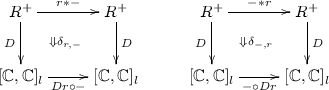## 5 Multicategory of Symmetric Lax Monoidal Multifunctors

Proposition 1 says that by fixing one index of the doubly-indexed natural transformation $$\delta _{-,=}:D({-}*{=})\rightarrow D{-}\circ D{=}$$, we obtain a 2-cell in the double category $$\mathbf {SMC}$$. However, $$\delta$$ itself does not live in $$\mathbf {SMC}$$. In order to create a room to accommodate $$\delta$$ as a kind of 2-cell, we extend horizontal morphisms of $$\mathbf {SMC}$$ to multi-ary functors that are symmetric lax monoidal in each argument. We first study such multi-ary functors in this section.

Let $$\mathbb {C}_i$$ ($$1\le i\le n)$$ and $$\mathbb {D}$$ be symmetric monoidal categories. Intuitively, an n-ary functor $$F:\mathbb {C}_1\times \cdots \times \mathbb {C}_n\rightarrow \mathbb {D}$$ is symmetric lax monoidal in each argument if it comes with a structure making the functor $$F(C_1,..,-_m,..,C_n):\mathbb {C}_m\rightarrow \mathbb {D}$$ symmetric lax monoidal for each $$m\in \{1,\cdots ,n\}$$ and $$C_i\in \mathbb {C}_i$$, $$i\in \{1,\cdots ,n\}\backslash \{m\}$$. Moreover, these symmetric lax monoidal structures commute with each other in a coherent manner.

To formally define such multi-ary symmetric lax monoidal functors, we introduce a notation for sequences. For a sequence $$C=C_1,\cdots ,C_n$$ of mathematical objects, a natural number $$1\le i\le n$$ and another sequence D, by C[i : D] we mean the sequence obtained by replacing $$C_i$$ with D. For instance, $$(1,3,5)[2:X,Y]=1,X,Y,5$$. When D is empty, C[i : ] stands for the sequence obtained by removing the i-th element of C.

### Definition 2

A symmetric lax monoidal multifunctor of type $$(\mathbb {C}_1,\cdots ,\mathbb {C}_n)\rightarrow \mathbb {D}$$ consists of a functor and a family of natural transformations indexed by $$1\le i\le n$$:

\begin{aligned} F&:\mathbb {C}_1\times \cdots \times \mathbb {C}_n\rightarrow \mathbb {D}\\ \phi ^i_{C[i:]}&:\mathbf {I}\rightarrow F(C[i:\mathbf {I}])\quad (C\in \mathbb {C}_1\times \cdots \times \mathbb {C}_n) \\ \phi ^i_{C[i:X,Y]}&:F(C[i:X])\otimes F(C[i:Y])\rightarrow F(C[i:X\otimes Y])\quad (C\in \mathbb {C}_1\times \cdots \times \mathbb {C}_n,X,Y\in \mathbb {C}_i) \end{aligned}

such that:

1. 1.

For each $$C\in \mathbb {C}_1\times \cdots \times \mathbb {C}_n$$ and $$1\le i\le n$$, The tuple $$(F(C[i:-]),$$$$\phi ^i_{C[i:]},\phi ^i_{C[i:-,=]})$$ is a symmetric lax monoidal functor from $$\mathbb {C}_i$$ to $$\mathbb {D}$$. We denote it by F(C / i).

2. 2.

The following equalities hold for each $$C\in \mathbb {C}_1\times \cdots \times \mathbb {C}_n$$ and $$1\le i< j\le n$$:

• $$\phi ^i_{C[j:\mathbf {I}][i:]} = \phi ^j_{C[i:\mathbf {I}][j:]}$$

• $$\phi ^j_{C[i:\mathbf {I}][j:P,Q]}\circ (\phi ^i_{C[j:P][i:]}\otimes \phi ^i_{C[j:Q][i:]}) = \phi ^i_{C[j:P\otimes Q][i:]}\circ \iota$$

• $$\phi ^i_{C[j:\mathbf {I}][i:P,Q]}\circ (\phi ^j_{C[i:P][j:]}\otimes \phi ^j_{C[i:Q][j:]}) = \phi ^j_{C[i:P\otimes Q][j:]}\circ \iota$$

• $$\phi ^j_{C[i:X\otimes Y][j:P,Q]}\circ (\phi ^i_{C[j:P][i:X,Y]}\otimes \phi ^i_{C[j:Q][i:X,Y]})=\phi ^i_{C[j:P\otimes Q][i:X, Y]}\circ (\phi ^j_{C[i:X][j:P,Q]}\otimes \phi ^j_{C[i:Y][j:P,Q]})\circ \tau$$.

We note that a symmetric lax monoidal multifunctor of type $$()\rightarrow \mathbb {D}$$ is just an object in $$\mathbb {D}$$, because all natural transformations vanish and only the functor of type $$1\rightarrow \mathbb {D}$$ remains.

### Example 4

Let us see how the definition of a binary symmetric lax monoidal multifunctor $$M:(\mathbb {C},\mathbb {C})\rightarrow \mathbb {C}$$ is unfolded. It consists of a functor $$M:\mathbb {C}\times \mathbb {C}\rightarrow \mathbb {C}$$ and the following natural transformations:

\begin{aligned}&\phi ^1_C:\mathbf {I}\rightarrow M(\mathbf {I},C),\quad \phi ^1_{X,Y,C}:M(X,C)\otimes M(Y,C)\rightarrow M(X\otimes Y,C) \\&\phi ^2_C:\mathbf {I}\rightarrow M(C,\mathbf {I}),\quad \phi ^2_{C,X,Y}:M(C,X)\otimes M(C,Y)\rightarrow M(C,X\otimes Y) \end{aligned}

such that

1. 1.

For each $$C\in \mathbb {C}$$, $$(M(-,C),\phi ^1_C,\phi ^1_{-,=,C})$$ and $$(M(C,-),\phi ^2_C,\phi ^2_{C,-,=})$$ are symmetric lax monoidal functors of type $$\mathbb {C}\rightarrow \mathbb {C}$$.

2. 2.

The following coherence axioms holds:

\begin{aligned}&\phi ^1_\mathbf {I}=\phi ^2_\mathbf {I}, \quad \phi ^1_{C\otimes C'}\circ \iota =\phi ^2_{\mathbf {I},C,C'}\circ (\phi ^1_C\otimes \phi ^1_{C'}),\quad \phi ^2_{C\otimes C'}\circ \iota =\phi ^1_{C,C',\mathbf {I}}\circ (\phi ^2_C\otimes \phi ^2_{C'}) \\&\phi ^2_{C\otimes C',D,D'}\circ (\phi ^1_{C,C',D}\otimes \phi ^1_{C,C',D'}) = \phi ^1_{C, C',D\otimes D'}\circ (\phi ^2_{C,D,D'}\otimes \phi ^2_{C',D,D'})\circ \tau \end{aligned}

We will later use the following binary symmetric lax monoidal multifunctors. Let R be a partially ordered semiring and $$\mathbb {C}$$ be a symmetric monoidal category.

1. 1.

The multiplication $$(*)$$ is a symmetric lax monoidal multifunctor of type $$(R^+,R^+)\rightarrow R^+$$.

2. 2.

The evaluation functor $$ev:[\mathbb {C},\mathbb {C}]_l\times \mathbb {C}\rightarrow \mathbb {C}$$ extends to a symmetric lax monoidal multifunctor of type $$([\mathbb {C},\mathbb {C}]_l,\mathbb {C})\rightarrow \mathbb {C}$$.

3. 3.

The functor composition $$(\circ )$$ extends to a symmetric lax monoidal multifunctor of type $$([\mathbb {C},\mathbb {C}]_l,[\mathbb {C},\mathbb {C}]_l)\rightarrow [\mathbb {C},\mathbb {C}]_l$$.

Note that $$(*)$$ is symmetric strict monoidal in each argument, while $$(\circ ), ev$$ are symmetric strict monoidal in the first argument, and symmetric lax monoidal in the second argument.

Next, for symmetric lax monoidal multifunctors $$(F,\phi ):(\mathbb {C}_1,\cdots ,\mathbb {C}_n)\rightarrow \mathbb {D}$$ and $$(G_i,\gamma (i)):(\mathbb {B}_{i,1},\cdots ,\mathbb {B}_{i,m_i})\rightarrow \mathbb {C}_i$$ ($$1\le i\le n$$), we define their multi-composition. First, we define a bijection $$(/):\{(i,j)~|~1\le i\le n,1\le j\le m_i\}\rightarrow \{1,\cdots ,\sum _{1\le i\le n}m_i\}$$, and represent a number in the latter set as the pair of numbers uniquely determined by ( / ) in the former set. Then the multicomposition is given by the following $$(H,\eta )$$:

\begin{aligned} H&=F\circ (G_1\times \cdots \times G_n) \\ \eta ^{i/j}_{(B_1,\cdots ,B_n)[i/j:]}&= F((GB_1,\cdots ,GB_n)[i:\gamma (i)^j_{B_i[j:]}]\circ \phi ^i_{(GB_1,\cdots ,GB_n)[i:]}\\ \eta ^{i/j}_{(B_1,\cdots ,B_n)[i/j:X,Y]}&= F((GB_1,\cdots ,GB_n)[i:\gamma (i)^j_{B_i[j:X,Y]}]\circ \phi ^i_{(GB_1,\cdots ,GB_n)[i:G(B_i[j:X]),G(B_i[j:Y])]} \end{aligned}

### Theorem 3

Symmetric monoidal categories, symmetric lax monoidal multifunctors, and the above multi-composition form a multicategory $$\mathbf {MSMC}_l$$.

### Proof

(Proof sketch). To check that symmetric lax monoidal multifunctors are closed under multicomposition, the key case is when $$n=2,m_1=m_2=1$$ and $$n=1,m_1=2$$.

In $$\mathbf {MSMC}_l$$ we consider monoids and monoid actions. A monoid is a tuple $$(\mathbb {C},U:()\rightarrow \mathbb {C}, M:(\mathbb {C},\mathbb {C})\rightarrow \mathbb {C})$$ of a symmetric monoidal category $$\mathbb {C}$$ and symmetric lax monoidal multifunctors UM such that

\begin{aligned} \mathrm{Id}=M\circ (\mathrm{Id},U),\quad \mathrm{Id}=M\circ (U,\mathrm{Id}),\quad M\circ (\mathrm{Id},M)=M\circ (M,\mathrm{Id}). \end{aligned}

An action of a monoid $$(\mathbb {C},U,M)$$ on a symmetric monoidal category $$\mathbb {D}$$ is a symmetric lax monoidal multifunctor $$A:(\mathbb {C},\mathbb {D})\rightarrow \mathbb {D}$$ such that

\begin{aligned} A\circ (U,\mathrm{Id})=\mathrm{Id},\quad A\circ (\mathrm{Id},A)=A\circ (M,\mathrm{Id}). \end{aligned}

By unfolding the definition, a monoid $$(\mathbb {C},U,M)$$ in $$\mathbf {MSMC}_l$$ equips $$\mathbb {C}$$ with an additional strict monoidal structure (UM). The argument-wise symmetric lax monoidal structure on M becomes a lax distributivity (see Example 4). Thus we call a monoid in $$\mathbf {MSMC}_l$$ a lax distributive strict rig category. It has a smaller set of coherence axioms than the one given by Laplaza in , thanks to the strictness of (UM).

### Example 5

(Continued from Example 4). $$(R^+,1,*)$$ and $$([\mathbb {C},\mathbb {C}]_l,\mathrm{Id},\circ )$$ are both lax distributive strict rig categories. Both monoids acts on themselves. The latter monoid acts on $$\mathbb {C}$$ with the evaluation functor ev.

We now extend the double category $$\mathbf {SMC}$$ of Grandis and Paré by replacing horizontal morphisms with symmetric lax monoidal multifunctors. The concept of 2-cells in $$\mathbf {SMC}$$ is also replaced by prisms — the reason of the name is because they are placed in the middle of the space surrounded by two horizontal multifunctors and vertical morphisms. Such a prism is defined to be a natural transformation that is a 2-cell of $$\mathbf {SMC}$$ in each argument.

### Definition 3

Let $$F:(\mathbb {C}_1,\cdots ,\mathbb {C}_n)\rightarrow \mathbb {D}$$ and $$G:(\mathbb {E}_1,\cdots ,\mathbb {E}_n)\rightarrow \mathbb {F}$$ be symmetric lax monoidal multifunctors and $$V_i:\mathbb {C}_i\rightarrow \mathbb {E}_i$$ ($$1\le i\le n$$) and $$W:\mathbb {D}\rightarrow \mathbb {F}$$ be symmetric colax monoidal functors. A prism $$\alpha$$ of type $$(V_1,\cdots ,V_n)\rightarrow W:F\rightarrow G$$, which is depicted asis a natural transformation $$\alpha :W\circ F\rightarrow G\circ (V_1\times \cdots \times V_n)$$ such that for each $$C\in \prod _{i=1}^n\mathbb {C}_i$$ and $$1\le i\le n$$, $$\alpha _{C[i:-]}$$ is a 2-cell of the following type in the double category $$\mathbf {SMC}$$: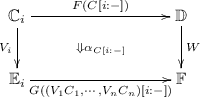We note that when $$n=0$$, a prism $$\alpha :()\rightarrow W:F\rightarrow G$$ is simply a morphism $$\alpha :WF\rightarrow G$$ in $$\mathbb {F}$$.

### Proposition 2

Let $$D:R^+\rightarrow [\mathbb {C},\mathbb {C}]_l$$ be a symmetric colax monoidal functor and $$\delta$$ be a prism of type $$(D,D)\rightarrow D:(*)\rightarrow (\circ )$$, where $$(*)$$ and $$(\circ )$$ are symmetric lax monoidal multifunctors appeared in Example 4. Then for each $$r\in R$$, $$\delta _{r,-}$$ and $$\delta _{-,r}$$ are 2-cells of the following type in $$\mathbf {SMC}$$: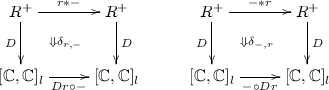Like double categories, composition of prisms can be done in two directions. Consider the following prisms ($$1\le i\le n$$).

Then define vertical composition and horizontal multicomposition of prisms by the following (ordinary) natural transformations:

\begin{aligned} \beta \circledcirc \alpha&=(\beta \circ (V_1\times \cdots \times V_n))\bullet (W'\circ \alpha ) \\ \alpha \circledast (\gamma _1,\cdots ,\gamma _n)&=(F'\circ (\gamma _1\times \cdots \times \gamma _n))\bullet (\alpha \circ (G_1\times \cdots \times G_n)) \end{aligned}

where $$\bullet$$ on the right hand side is the vertical composition of natural transformations.

### Proposition 3

In the above setting,

1. 1.

$$\beta \circledcirc \alpha$$ is a prism of type $$(V'_1\circ V_1,\cdots ,V'_n\circ V_n)\rightarrow W'\circ W: F\rightarrow F''$$.

2. 2.

$$\alpha \circledast (\gamma _1,\cdots ,\gamma _n)$$ is a prism of type $$(U_{1,1},\cdots ,U_{n,m_n})\rightarrow W:F\circ (G_1,\cdots ,G_n)\rightarrow F'\circ (G'_1,\cdots ,G'_n)$$.

3. 3.

The interchange law holds:

\begin{aligned} (\beta \circledast (\delta _1,\cdots ,\delta _n))\circledcirc (\alpha \circledast (\gamma _1,\cdots ,\gamma _n))= (\beta \circledcirc \alpha )\circledast (\delta _1\circledcirc \gamma _1,\cdots ,\delta _n\circledcirc \gamma _n). \end{aligned}

### Definition 4

Let $$(\mathbb {C},U,M),(\mathbb {D},U',M')$$ be monoids in $$\mathbf {MSMC}_l$$. A vertical monoid homomorphism consists of a symmetric colax monoidal functor $$A:\mathbb {C}\rightarrow \mathbb {D}$$ and prisms $$\epsilon :()\rightarrow A:U\rightarrow U'$$ and $$\delta :(A,A)\rightarrow A:M\rightarrow M'$$:

such that the following prism equalities hold:

\begin{aligned}&\delta \circledast (\mathrm{id},\epsilon )=\mathrm{id},\quad \delta \circledast (\epsilon ,\mathrm{id})=\mathrm{id},\quad \delta \circledast (\mathrm{id},\delta )=\delta \circledast (\delta ,\mathrm{id}). \end{aligned}

The above prism equalities amounts to the following equality of natural transformations:

\begin{aligned}&M'(AX,\epsilon )\circ \delta _{X,U}=\mathrm{id}\quad M'(\epsilon ,AX)\circ \delta _{U,X}=\mathrm{id}\\&M'(AX,\delta _{Y,Z})\circ \delta _{X,M(Y,Z)} = M'(\delta _{X,Y},AZ)\circ \delta _{M(X,Y),Z} \end{aligned}

### Theorem 4

There is a bijective correspondence between

1. 1.

A vertical monoid homomorphism $$(D,\epsilon ,\delta )$$ from $$(R^+,1,*)$$ to $$([\mathbb {C},\mathbb {C}]_l,\mathrm{Id},\circ )$$.

2. 2.

An R-graded linear exponential comonad on $$\mathbb {C}$$.

Vertical monoid homomorphisms vertically compose. Therefore we can extend a graded linear exponential comonad (as a vertical monoid homomorphism) by stacking vertical monoid homomorphisms.

### Proposition 4

Let RS be partially ordered semirings. Then a vertical monoid homomorphism from $$(R^+,1_R,*_R)$$ to $$(S^+,1_S,*_S)$$ bijectively corresponds to a monotone function $$h:(R,\le _R)\rightarrow (S,\le _S)$$ such that $$h(\sum _R r_i)\le \sum _S h(r_i)$$ and $$h(\prod _R r_i)\le \prod _S h(r_i)$$ (which we call colax homomorphism).

### Proposition 5

Let $$F\dashv U:\mathbb {C}\rightarrow \mathbb {D}$$ be a symmetric lax monoidal adjunction. Then the functor $$V^{F\dashv U}$$ defined by $$V^{F\dashv U}H=F\circ H\circ U$$ is a vertical monoid homomorphism from $$([\mathbb {C},\mathbb {C}]_l,\mathrm{Id},\circ )$$ to $$([\mathbb {D},\mathbb {D}]_l,\mathrm{Id},\circ )$$.

### Proof

Let $$F\dashv U:\mathbb {C}\rightarrow \mathbb {D}$$ be a symmetric lax monoidal adjunction. From Kelly’s doctrinal adjunction, F is symmetric strong monoidal, hence so is $$F\circ -$$ in the following diagram:Next, $$-\circ U$$ above is always symmetric strict monoidal. By composing them, we obtain that $$V^{F\dashv U}$$ is symmetric strong, hence colax monoidal. We next introduce prisms $$(\epsilon ,\delta )$$ of the following type:We define $$\epsilon$$ to be the counit of the adjunction $$F\dashv U$$, which is monoidal natural, and $$\delta$$ be the following natural transformation:

\begin{aligned} \delta _{H_1,H_2}=V^{F\dashv U}(H_1\circ \eta \circ H_2):V^{F\dashv U}(H_1\circ H_2)\rightarrow V^{F\dashv U}H_1\circ V^{F\dashv U}H_2 \end{aligned}

It is routine to check that this satisfies the axioms of prism.    $$\square$$

### Theorem 5

Let R be a partially ordered semiring and D be an R-graded linear exponential comonad on a symmetric monoidal category $$\mathbb {C}$$. We moreover let S be another partially ordered semiring, $$h:S\rightarrow R$$ be a colax homomorphism and $$F\dashv U:\mathbb {C}\rightarrow \mathbb {D}$$ be a symmetric lax monoidal adjunction. Then the following composite of vertical monoid homomorphisms is an S-graded linear exponential comonad on $$\mathbb {D}$$.

We call the above composite the extension of D with $$F\dashv U$$ and h.

## 7 From Monoid Actions to Graded Comonoid-Coalgebras

Let $$(D,\epsilon ,\delta ):(R^+,1,*)\rightarrow ([\mathbb {C},\mathbb {C}]_l,\mathrm{Id},\circ )$$ be an R-graded linear exponential comonad as a vertical monoid homomorphism. The prism equations in Definition 4 suggests that the vertical monoid homomorphism itself can be seen as a monoid. We can thus consider monoid actions of $$(D,\epsilon ,\delta )$$: it consists of a prism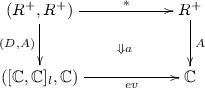such that the following prism equations hold:

\begin{aligned} a\circledast (\delta ,\mathrm{id})=a\circledast (\mathrm{id},a),\quad a\circledast (\epsilon ,\mathrm{id})=\mathrm{id}. \end{aligned}

We note that this makes sense because $$(*)$$ and ev are also monoid actions in $$\mathbf {MSMC}_l$$; see Example 5. By unfolding this definition, we obtain the following structure, which we name graded comonoid-coalgebra.

### Definition 5

Let R be a partially ordered semiring. An R-graded comonoid-coalgebra of an R-graded linear exponential comonad $$(D,w,c,\epsilon ,\delta )$$ on a symmetric monoidal category $$\mathbb {C}$$ is a tuple (Aauo) such that

• $$(A,u,o):R^+\rightarrow \mathbb {C}$$ is a symmetric colax monoidal functor.

• $$a_{r,r'}:A(r*r')\rightarrow D (r) (A(r'))$$ is a natural transformation.

They satisfy the following six equational axioms:

A morphism from an R-graded comonoid-coalgebra (Aauo) to another (Bbvp) is a monoidal natural transformation $$h:(A,u,o)\rightarrow (B,v,p)$$ such that h satisfies: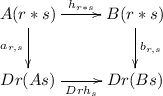We write $$C(\mathbb {C},D)$$ for the category of R-graded comonoid-coalgebras of D.

### Proposition 6

Let R be a partially ordered semiring and $$(D,w,c,\epsilon ,\delta )$$ be an R-graded linear exponential comonad on a symmetric monoidal category $$\mathbb {C}$$. The following gives a symmetric monoidal structure on $$C(\mathbb {C},D)$$:

\begin{aligned}&\mathbf {I} =(\dot{\mathbf {I}}, (\lambda {r,s}~.~m_r), \mathrm{id}_\mathbf {I}, (\lambda {r,s}~.~\iota ^{-1})) \\&(A,a,u,o)\otimes (B,b,v,p)\\&=(A\mathbin {\dot{\otimes }}B,~~\lambda {r,r'}~.~m_{r,Ar',Br'}\circ (a_{r,r'}\otimes b_{r,r'}),~~ \iota \circ (u\otimes v),~~\lambda {r,r'}~.~\tau \circ (o_{r,r'}\otimes p_{r,r'})) \\&(f\otimes g)_r=f_r\otimes g_r \\&(\lambda _A)_r =\lambda _{Ar},\quad (\rho _A)_r =\rho _{Ar},\quad (\alpha _{A,B,C})_r =\alpha _{Ar,Br,Cr},\quad (\sigma _{A,B})_r=\sigma _{Ar,Br} \end{aligned}

When $$R=1$$, The category $$C(\mathbb {C},D)$$ reduces to the category of Eilenberg-Moore coalgebras of the non-graded linear exponential comonad.

### Theorem 6

Let $$(D,w,c,\epsilon ,\delta )$$ be a 1-graded linear exponential comonad on a symmetric monoidal category $$\mathbb {C}$$. Then the category $$C(\mathbb {C},D)$$ is strong monoidally isomorphic to the category $$\mathbb {C}^D$$ of Eilenberg-Moore coalgebras of the comonad $$(D,\epsilon ,\delta )$$.

Like $$\mathbb {C}^D$$, there is a symmetric lax monoidal adjunction of the following type: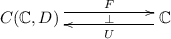but this itself is not enough to recover DD takes two arguments, while the composite $$F\circ U$$ is only equal to the symmetric lax monoidal comonad D1 on $$\mathbb {C}$$. The category $$C(\mathbb {C},D)$$ actually carries an R-twist T, which acts on comonoid-coalgebras as follows:

\begin{aligned} Tr(A,\cdots )=(A(-*r),\cdots ), \end{aligned}

and D is recovered as the extension of T with the adjunction $$F\dashv U$$ (Theorem 5).

### Theorem 7

Let R be a partially ordered semiring and $$(D,w,c,\epsilon ,\delta )$$ be an R-graded linear exponential comonad on a symmetric monoidal category $$\mathbb {C}$$.

1. 1.

The functor $$F:C(\mathbb {C},D)\rightarrow \mathbb {C}$$ given by $$F(A,a,u,o)=A1$$ and $$Fh=h_1$$ is symmetric strict monoidal, and has a symmetric lax monoidal right adjoint $$U:\mathbb {C}\rightarrow C(\mathbb {C},D)$$, whose object part is given by $$UA=(\lambda { r}\,.\, DrA, \lambda {r,r'}\, .\, \delta _{r,{r'},A},w_{A},$$$$\lambda {r,r'}\, .\, c_{r,{r'},A})$$.

2. 2.

The following data give an R-twist T on $$C(\mathbb {C},D)$$:

\begin{aligned}&TrA=(\lambda {s}~.~A(s*r),~~\lambda {s,s'}~.~a_{s,s'*r},~~u,~~\lambda {s,s'}~.~o_{s*r,s'*r}),\quad (Tr h)_t=h_{t*r}\\&(m^T_r)_t=\mathrm{id}_\mathbf {I},\quad (m^T_{r,A,B})_t=\mathrm{id}_{A(t*r)\otimes B(t*r)},\quad (w^T_{A})_t=u,\quad (c^T_{r,s,A})_t=o_{t*r,t*s}. \end{aligned}

Here, $$A=(A,a,u,o)$$ and B are R-graded comonoid coalgebras. From the definition of twists, $$\epsilon ^T,\delta ^T$$ are identities.

3. 3.

The extension of D with $$F\dashv U$$ (Theorem 5) coincides with the R-graded linear exponential comonad D.

The following classic result [1, Theorem 6-1] can be reproved by Theorem 7.

### Corollary 1

Let $$\mathbb {C}$$ be a symmetric monoidal category and Let D be a non-graded linear exponential comonad on $$\mathbb {C}$$. The canonical symmetric monoidal structure on the category $$\mathbb {C}^D$$ of Eilenberg-Moore coalgebras of D is cartesian.

### Proof

From Theorem 1, D is a 1-graded linear exponential comonad on $$\mathbb {C}$$. Therefore $$C(\mathbb {C},D)$$ has a 1-twist by Theorem 7-3. Therefore the symmetric monoidal structure of $$C(\mathbb {C},D)$$ is cartesian by Theorem 2. Finally, $$C(\mathbb {C},D)$$ is strong monoidally isomorphic to $$\mathbb {C}^D$$ by Theorem 6, hence the symmetric monoidal structure of $$\mathbb {C}^D$$ is also cartesian.    $$\square$$

We show the finality of the category of graded comonoid-coalgebras. Let R be a partially ordered semiring and D be an R-graded linear exponential comonad on a symmetric monoidal category $$\mathbb {C}$$. We define a resolution of D to be a pair of a symmetric lax monoidal adjunction $$J\dashv K:\mathbb {E}\rightarrow \mathbb {C}$$ and an R-twist $$(S,w^S,c^S)$$ on $$\mathbb {E}$$ such that the extension of S with $$J\dashv K$$ is equal to D. Then the following set of data becomes a strong monoidal functor $$(M,m^M,m^M_{E,E'}):\mathbb {E}\rightarrow C(\mathbb {C},D)$$:

\begin{aligned}&ME= (\lambda {r}~.~J(Sr)E,~~ \lambda {r,r'}~.~J(Sr)\eta ^{J\dashv K}_{Sr'E},~~ (m^J)^{-1}\circ w^S_E,~~ \lambda {r,r'}~.~(m^J_{SrE,Sr'E})^{-1}\circ Jc^S_{r,r',E}) \\&(Mf)_r=J(Sr)f,\quad (m^M)_r=J(m^S_r)\circ m^J,\quad (m^M_{E,E'})_r=J(m^S_{r,E,E'})\circ m^J_{SrE,SrE'} \end{aligned}

(recall that SrJ are both symmetric strong monoidal).

### Theorem 8

The above M is the unique symmetric strong monoidal functor such that:

1. 1.

Equality of symmetric lax monoidal functors $$M\circ K=U$$ and $$F\circ M=J$$ hold.

2. 2.

Let $$M^*=-\circ M$$ and $$M_*=M\circ -$$ be induced symmetric strict (resp. strong) monoidal functors. Then the following square of symmetric colax monoidal functors commutes.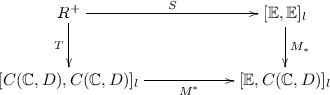## 8 Conclusion

We have given a concise characterization of graded linear exponential comonad as a vertical monoid homomorphism $$(D,\epsilon ,\delta )$$ from $$(R^+,1,*)$$ to $$([\mathbb {C},\mathbb {C}]_l,\mathrm{Id},\circ )$$. This characterization is built upon a combination of the theory of symmetric lax monoidal multifunctors and Grandis and Paré’s double category of symmetric monoidal categories. After this characterization, we considered monoid actions, and derived the concept of graded comonoid-coalgebras. The category of graded comonoid-coalgebras are shown to give a resolution of the graded linear exponential comonad D. These results are consistent with the theory of non-graded linear exponential comonads developed in .

It remains to be seen if the category of graded comonoid-coalgebras can be constructed in a purely double-category theoretic way. In non-graded case, there are other type of categorical models of exponential modality using Lafont category and Seely category . Graded version of these categories are also an interesting research topic.